# Affine Interpretation of the BS-Warps

#### Notation.

In this chapter, we denote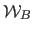the BS-Warp. As a reminder of section 2.3.2, a BS-Warp is defined as: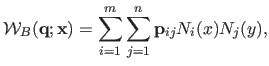(6.2)

where the functionsare the B-Spline basis functions and theare the control points (grouped in the parameter vector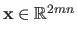). The scalars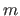andare the number of control points along theand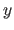directions respectively. Note that we consider as coincident the knot sequences used to define the B-Spline basis functions.

#### Affine interpretation.

If we consider that the observed surface is modelled by a threedimensional tensor-product B-Spline, the BS-Warp corresponds to the transformation between the two images under affine imaging conditions (see figure 6.1 for an illustration).Let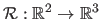be the 2D-3D map between the first image and the threedimensional surface:(6.3)

withthe 3D control points of the surface. Let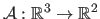be the affine projection of the surface into the second image:(6.4)

withthe matrix which models the affine projection, assuming that the 3D surface is expressed within the coordinate frame of the second camera: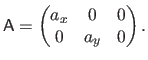(6.5)

Given these notations, the warped pointcan be written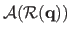which, after expansion, gives: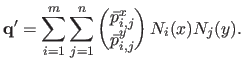(6.6)

Equation (6.6) matches the definition of equation (6.2) of a BS-Warp.

#### BS-Warps are not suited for perspective imaging conditions.

As we just demonstrated, BS-Warps are obtained under affine imaging conditions. However, this does not prove that they are not suited for perspective imaging conditions. In this paragraph, we experimentally illustrate that BS-Warps are indeed not suited for perspective imaging conditions. This comes from the fact that the division appearing in a perspective projection is not present in the BS-Warp model.

The bad behavior of the BS-Warp in the presence of perspective effects is illustrated in figure 6.2. In this experiment, we simulate a set of point correspondences by transforming a regular grid with an homography parametrized by a scalar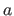that controls the amount of perspective effect. The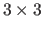matrix of this homography,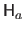, is given by: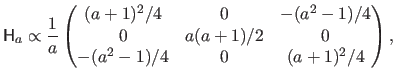(6.7)

where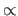indicates equality up to scale. The larger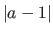, the more important the perspective effect. Figure 6.2 c clearly shows that the transformation can be correctly modelled by a BS-Warp only when, i.e. when the perspective effect is barely existant. Figure 6.2 d shows that the number of control points of a BS-Warp must be significantly large in order to correctly model a perspective effect.
Figure 6.2: Bad behavior of the BS-Warp in the presence of perspective effects. (a) Data points on a regular grid (first image). (b) Transformed points simulating a perspective effect with an homography (second image). (c) Influence of the perspective effect on a BS-Warp with 16 control points. (d) The perspective effect () can be modeled with a BS-Warp but it requires a large amount of control points.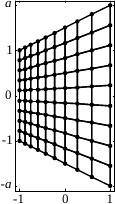(a) (b) (c) (d)

Contributions to Parametric Image Registration and 3D Surface Reconstruction (Ph.D. dissertation, November 2010) - Florent Brunet
Webpage generated on July 2011